﻿ 某型无人机群的监视覆盖任务航路规划

# 某型无人机群的监视覆盖任务航路规划Route Planning of Surveillance Coverage Mission for a Certain Type of UAV Group

Abstract: Using drones to carry out surveillance tasks has a high efficiency cost ratio in border and regional control, anti-terrorism and explosion-proof surveillance and military applications. The route planning algorithm of UAV group surveillance coverage is the core algorithm to improve the efficiency and capability of UAV group surveillance task. The traditional coverage route planning algorithm results in a single style, poor flexibility in the adversarial environment, and the area division method is not convenient for automatic computer generation. In this paper, a surveillance coverage route planning algorithm based on artificial potential field and genetic algorithm is proposed to generate a variety of surveillance coverage routes with good adversary in the execution of surveillance tasks. On the basis of the artificial potential field method, the seed of excitation potential field is encoded as a gene in the form of binary string, and the diversity of seed pattern is increased through the operation of crossover, mutation, merge and other operators, so as to plan a surveillance coverage route with fewer turns, short surveillance interval and good antagonism. Finally, an example is given to verify the algorithm, and the results show that the algorithm can effectively meet the needs of surveillance mission coverage route planning.

1. 引言

2. 区域划分方法

2.1. 任务区域网格化

$c=\sqrt{2}r$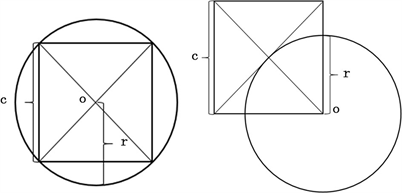Figure 1. Grid unit distance

2.2. 矩形任务区域划分

2.3. 不规则任务区域划分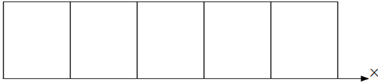Figure 2. Rectangle target area partition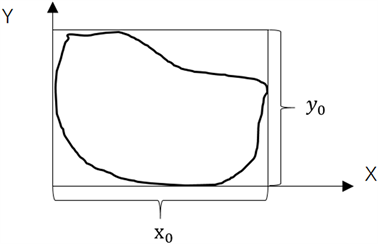Figure 3. Irregular target region

3. 监视覆盖航路规划问题建模

3.1. 人工势场法生成覆盖航路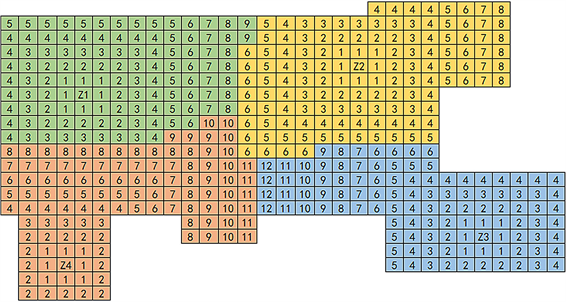Figure 4. Irregular division

3.2. 监视覆盖航路的目标函数

1) 转弯角度总值最小

${z}_{1}=\underset{i=1}{\overset{n}{\sum }}{q}_{i}$

2) 最大转弯角度

$|\mathrm{arctan}\left(\frac{{y}_{m}-{y}_{m-1}}{{x}_{m}-{x}_{m-1}}\right)|\le \theta$

3) 网格上的势的总值及标准差最小化

${Z}_{3}=\underset{i=n}{\sum }{m}_{i}$

$M=\frac{{Z}_{3}}{n}$

${Z}_{4}=\sqrt{\frac{{\left({m}_{1}-M\right)}^{2}+{\left({m}_{2}-M\right)}^{2}+\cdots +{\left({m}_{n}-M\right)}^{2}}{n}}$

4) 航路的可预测性要小

${Z}_{5}={T}^{-1}$

4. 基于遗传算法的监视覆盖航路规划算法

4.1. 基因编码

4.2. 交叉、变异产生新的基因

1) 交叉算子

2) 变异算子

3) 合并算子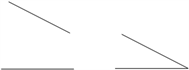Figure 5. Merge operation

4.3. 监视覆盖航路生成

5. 仿真实验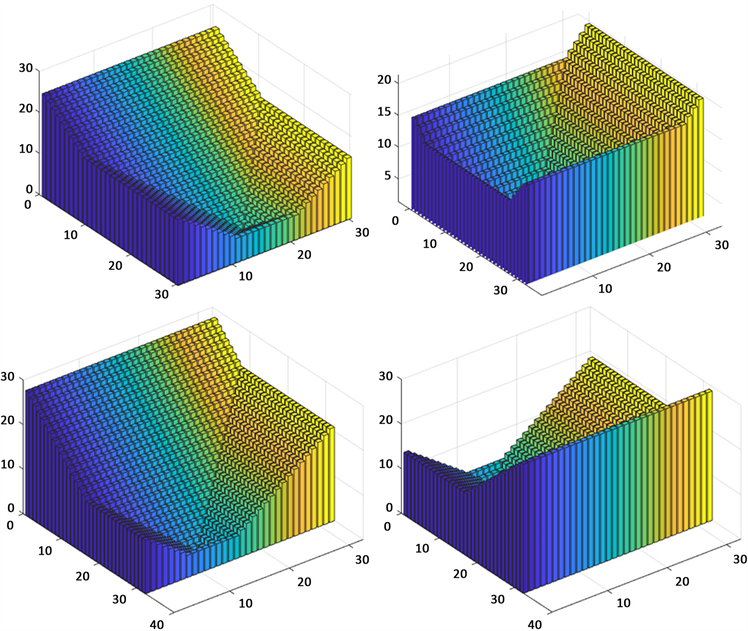Figure 6. Initial potential field

6. 小结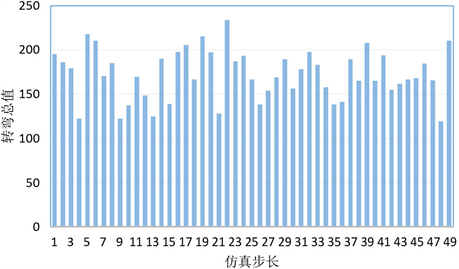Figure 7. Total turning angle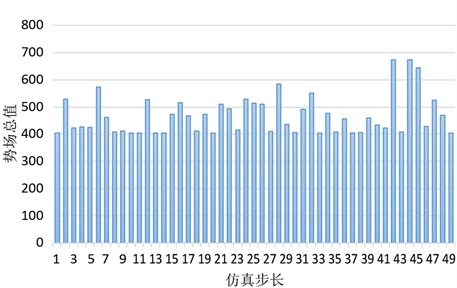Figure 8. Total potential field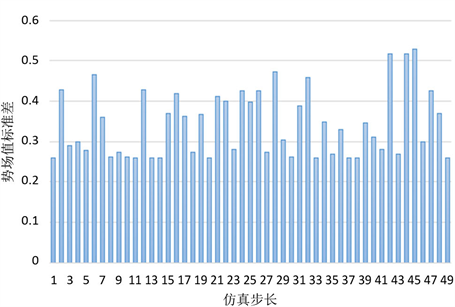Figure 9. Standard deviationFigure 10. Maximum grid potential

 李御驰, 闫军涛, 宋志华, 张晗. 基于遗传算法的无人机监视覆盖航路规划算法研究[J]. 计算机科学与应用, 2019, 9(6): 1208-1215.
https://doi.org/10.12677/csa.2019.96135

 李艳庆. 基于遗传算法和深度强化学习的多无人机协同区域监视的航路规划[D]: [硕士学位论文]. 西安: 西安电子科技大学, 2018.

 陈海, 王新民, 焦裕松, 李俨. 一种凸多边形区域的无人机覆盖航迹规划算法[J]. 航空学报, 2010, 31(9): 1802-1808.

 Acar, E.U., Choset, H., Rizzi, A.A., et al. (2002) Morse Decompositions for Coverage Tasks. The International Journal of Robotics Research, 21, 331-344.
https://doi.org/10.1177/027836402320556359

 Atkar, P., Greenfield, A.L., Conner, D.C., Choset, H. and Rizzi, A. (2005) Uniform Coverage of Automotive Surface Patches. The International Journal of Robotics Research, 24, 883-898.
https://doi.org/10.1177/0278364905059058

 Acar, E.U., Choset, H., Zhang, Y. and Schervish, M. (2003) Path Planning for Robotic Demining: Robust Sensor-Based Coverage of Un-structured Environments and Probabilistic Methods. International Journal of Robotics Research, 22, 441-466.
https://doi.org/10.1177/02783649030227002

 丁家如, 杜昌平, 赵耀, 等. 基于改进人工势场法的无人机路径规划算法[J]. 计算机应用, 2016, 36(1): 287-290.

 周明, 孙树栋. 遗传算法原理及应用[M]. 北京: 国防工业出版社, 1999.

 鱼佳欣, 周春来, 刘东平. 改进遗传算法的无人机航路规划与仿真[J]. 计算机仿真, 2013, 30(12): 17-20.

 贾广芝. 基于遗传算法和稀疏A*算法的无人机三维航迹规划研究[D]: [硕士学位论文]. 南京: 南京邮电大学, 2017.

Top